Test: Numerical solutions of linear & non-linear algebraic equations

# Test: Numerical solutions of linear & non-linear algebraic equations

Test Description

## 10 Questions MCQ Test Engineering Mathematics | Test: Numerical solutions of linear & non-linear algebraic equations

Test: Numerical solutions of linear & non-linear algebraic equations for Civil Engineering (CE) 2023 is part of Engineering Mathematics preparation. The Test: Numerical solutions of linear & non-linear algebraic equations questions and answers have been prepared according to the Civil Engineering (CE) exam syllabus.The Test: Numerical solutions of linear & non-linear algebraic equations MCQs are made for Civil Engineering (CE) 2023 Exam. Find important definitions, questions, notes, meanings, examples, exercises, MCQs and online tests for Test: Numerical solutions of linear & non-linear algebraic equations below.
Solutions of Test: Numerical solutions of linear & non-linear algebraic equations questions in English are available as part of our Engineering Mathematics for Civil Engineering (CE) & Test: Numerical solutions of linear & non-linear algebraic equations solutions in Hindi for Engineering Mathematics course. Download more important topics, notes, lectures and mock test series for Civil Engineering (CE) Exam by signing up for free. Attempt Test: Numerical solutions of linear & non-linear algebraic equations | 10 questions in 30 minutes | Mock test for Civil Engineering (CE) preparation | Free important questions MCQ to study Engineering Mathematics for Civil Engineering (CE) Exam | Download free PDF with solutions
 1 Crore+ students have signed up on EduRev. Have you?
Test: Numerical solutions of linear & non-linear algebraic equations - Question 1

### The iteration step in order to solve for the cube roots of a given number Nusing the Newton- Raphson’s method is

Detailed Solution for Test: Numerical solutions of linear & non-linear algebraic equations - Question 1

Concept:
Let x0 be an approximation root of f(x) = 0 and x1 = x0th be the correct root so that f(x1) = 0 expanding f(x0th) by Taylor's series we obtain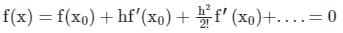Neglecting the second and higher order derivatives we have f(x0) + hf'(x0) = 0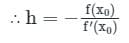A better approximation than x0 is therefore given by x1, where x1 = x0 + h =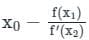successive approximations are given by x2, x3 ....xn+1 where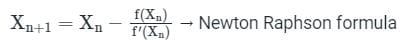For pth root of a given number N, is not of equation f(x) = xp - N = 0
Iteration equation: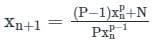for cube root, put p = 3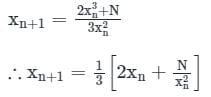Therefore, option (2) is correct one.

Test: Numerical solutions of linear & non-linear algebraic equations - Question 2

### The square root of a number N is to be obtained by applying the Newton Raphson iterations to the equation x2 - N = 0, if i denotes the iteration index, the correct iterative scheme will be

Detailed Solution for Test: Numerical solutions of linear & non-linear algebraic equations - Question 2

Given
Now,
f(x) = x2 – N = 0
Differentiating,
f’(x) = 2x
Now,
Using Newton-Raphson Method,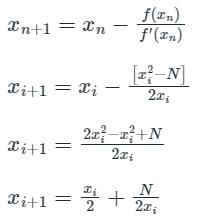xi+1 = (xi + N/xi)/2

Test: Numerical solutions of linear & non-linear algebraic equations - Question 3

### The Newton-Raphson method is said to have

Detailed Solution for Test: Numerical solutions of linear & non-linear algebraic equations - Question 3

Concept:
Newton- Raphson method:

• The Newton - Raphson method is the type of open method (Extrapolation method).
• It is a powerful technique for solving algebraic and transcendental equations f( x ) = 0, numerically.
• It is an iteration method for solving a set of various nonlinear equations with an equal number of unknowns.

• It possesses quadratic convergence characteristics. Therefore, the convergence is very fast.
• The number of iterations is independent of the size of the system.
• The Newton-Raphson Method convergence is not sensitive to the choice of slack bus.
• Overall, there is a saving in computation time since a fewer number of iterations are required.

• It does not converge to a root when the second differential coefficient changes sign
• It is sensitive to the starting value. Convergence fails if the starting point is not near the root.
• It is not preferred when the graph of f(x) is nearly horizontal where it crosses the x-axis as the values of f’(x) have negative values in this case.
Test: Numerical solutions of linear & non-linear algebraic equations - Question 4

If f(0) = 3, f(1) = 5, f(3) = 21, then the unique polynomial of degree 2 or less using Newton divided difference interpolation will be:

Detailed Solution for Test: Numerical solutions of linear & non-linear algebraic equations - Question 4

Concept:
Newton’s divided difference polynomial method:
Second order polynomial interpolation using Newton’s divided difference polynomial method is as follows,
Given (x0,y0), (x1,y1), (x2,y2) be the data points and f(x) be the quadratic interpolant, then f(x) is given by
f(x) = b0 + b1(x – x0) + b2 (x – x0)(x – x1);
Where
b0 = f(x0);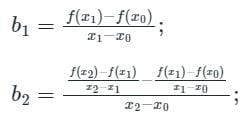Calculation:
Given f(0) = 3, f(1) = 5, f(3) = 21;
⇒ (0,3), (1,5), (3,21) are the data points;
The polynomial will be f(x) = b0 + b1(x) + b2 (x)(x – 1);
⇒ b0 = f(0) = 3;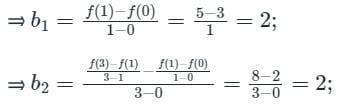Substituting the constant b0, b1, b2 in the quadratic interpolant,
⇒ f(x) = 3 + 2x + 2 (x)(x – 1) = 3 + 2x + 2x2 – 2x = 3 + 2x2;
The unique polynomial of degree 2 will be f(x) = 3 + 2x2;
Easy method:
To save time, simply substitute the data points in the polynomials given in options and find the polynomial that is satisfying all data points.

Test: Numerical solutions of linear & non-linear algebraic equations - Question 5

The iteration formula to find the reciprocal of a given number N by Newton’s method is

Detailed Solution for Test: Numerical solutions of linear & non-linear algebraic equations - Question 5

Concept:
Newton-Raphson method: It has order of convergence 2 and number of guesses required is 1.
Iteration formula,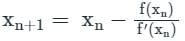Calculation: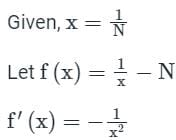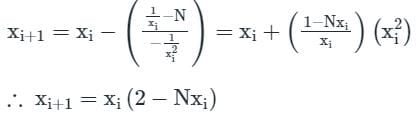Test: Numerical solutions of linear & non-linear algebraic equations - Question 6

The real root of x3 + x2 + 3x + 4 = 0 correct to four decimal places, obtained using Newton Raphson method is

Detailed Solution for Test: Numerical solutions of linear & non-linear algebraic equations - Question 6

Concept:
Newton-Raphson Method:
The iteration formula is given by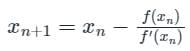Where x0 is the initial value/root of the equation f(x) = 0
Given,
f(x) = x3 + x2 + 3x + 4 = 0
f'(x) = 3x2 + 2x + 3
∴ f(-1) = 1 > 0 and f(-2) = -6 < 0
∴ f(-1).f(-2) < 0
⇒ ∃ a root lies in [-1, -2]
Let, x0 = -1
By Newton Raphson method
First approximation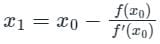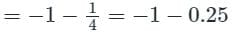x1 = -1.25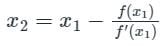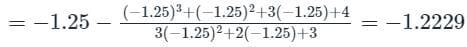Test: Numerical solutions of linear & non-linear algebraic equations - Question 7

To solve the equation 2 sin x = x by Newton-Raphson method, the initial guess was chosen to be x = 2.0. Consider x in radian only. The value of x (in radian) obtained after one iteration will be closest to

Detailed Solution for Test: Numerical solutions of linear & non-linear algebraic equations - Question 7

Concept:
The iterative formula for Newton Raphson method is given as,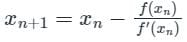[NOTE: Take the trignometric terms in Radian while using scitific calculator for this type of numericals]
Calculation:
Given:
f(x) = 2 sin x - x
∴ f'(x) = 2 cos x - 1
Initial guess is x0 = 2.0
The first iteration by Newton Raphson method is given by,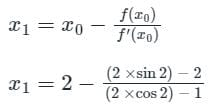⇒ x1 = 1.901

Test: Numerical solutions of linear & non-linear algebraic equations - Question 8

The order of convergence of Newton Raphson method is

Detailed Solution for Test: Numerical solutions of linear & non-linear algebraic equations - Question 8

Order of convergence of the Newton Raphson method is two
Important Point
Order of convergence of various numerical methods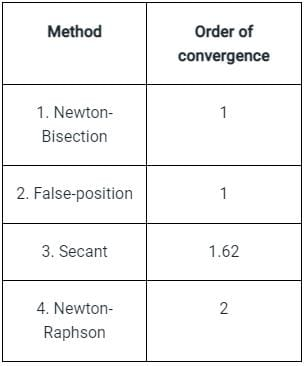Test: Numerical solutions of linear & non-linear algebraic equations - Question 9

The 2nd approximation to a root of the equation x2 - x - 1 = 0 in the interval (1, 2) by Bisection method will be:

Detailed Solution for Test: Numerical solutions of linear & non-linear algebraic equations - Question 9

Concept:
Bisection method:
Used to find the root for a function. Root of a function f(x) = a such that f(a)= 0
Property: if a function f(x) is continuous on the interval [a…b] and sign of f(a) ≠  sign of f(b). There is a value c belongs to [a…b] such that f(c) = 0, means c is a root in between [a….b]
Note:
Bisection method cut the interval into 2 halves and check which half contains a root of the equation.
1) Suppose interval [a…b] .
2) Cut interval in the middle to find m : m = (a+b)/2
3) sign of f(m) not matches with f(a) proceed the search in the new interval.
Calculation:
Given:
f(x) = x2 - x - 1 = 0  , a = 1 , b = 2
f(1) = 1 - 1 -1 = -1 < 0 , f(2) = 22 - 2 - 1 = 1 > 0 , Hence root lies between 1 and 2
By Bi-section method,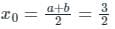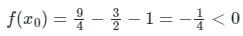Which is positive. Hence, the root lies between 1.5 and 2
By Bi-section method,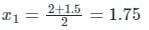The 2nd approximation to a root of the equation x2 - x - 1 = 0 in the interval (1, 2) by Bisection method is 1.75.

Test: Numerical solutions of linear & non-linear algebraic equations - Question 10

The approximate value of a root of x3 – 13 = 0, then 3.5 as initial value, after one iteration using Newton-Raphson method, is

Detailed Solution for Test: Numerical solutions of linear & non-linear algebraic equations - Question 10

Concept:
Newton-Raphson Method:
The iteration formula is given by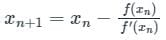Where x0 is the initial value/root of the equation f(x) = 0
Calculation:
Given:
f(x) = x3 - 13, x0 = 3.5
f'(x) = 3x2
f(x0) = f(3.5) = 3.53 - 13 = 29.875
f'(x0) = f'(3.5) = 3 × 3.52 = 36.75
We know that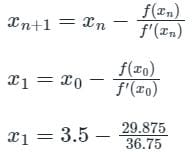∴ x1 = 2.6871

## Engineering Mathematics

47 videos|119 docs|75 tests
Information about Test: Numerical solutions of linear & non-linear algebraic equations Page
In this test you can find the Exam questions for Test: Numerical solutions of linear & non-linear algebraic equations solved & explained in the simplest way possible. Besides giving Questions and answers for Test: Numerical solutions of linear & non-linear algebraic equations, EduRev gives you an ample number of Online tests for practice

## Engineering Mathematics

47 videos|119 docs|75 tests(Scan QR code)